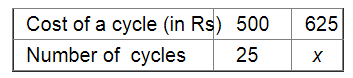# A person has money to buy 25 cycles worth Rs 500 each.`
Question:

A person has money to buy 25 cycles worth Rs 500 each. How many cycles he will be able to buy if each cycle is costing Rs 125 more?

Solution:

Let x be the number of cycles bought if each cycle costs Rs 125 more.It is in inverse variation. Therefore, we get:

$500 \times 25=625 \times x$

$\Rightarrow x=\frac{500 \times 25}{625}$

$500 \times 25=625 \times x$

$\Rightarrow x=\frac{500 \times 25}{625}$

$=20$

$\therefore$ The required number of cycles is 20 .